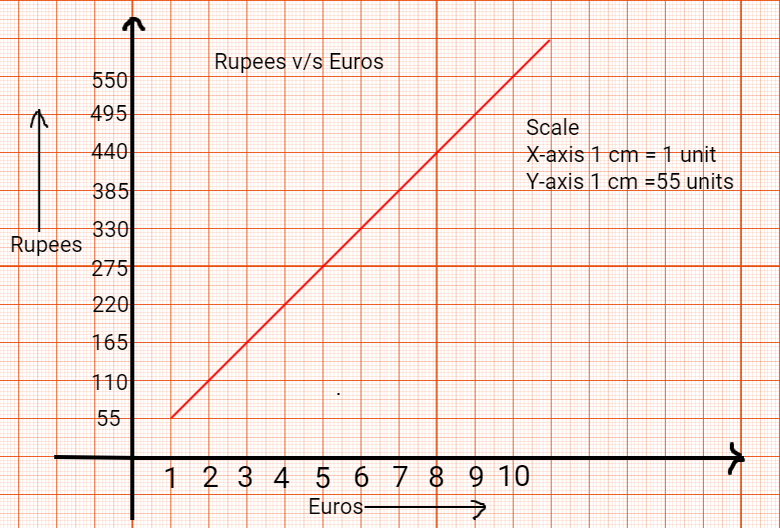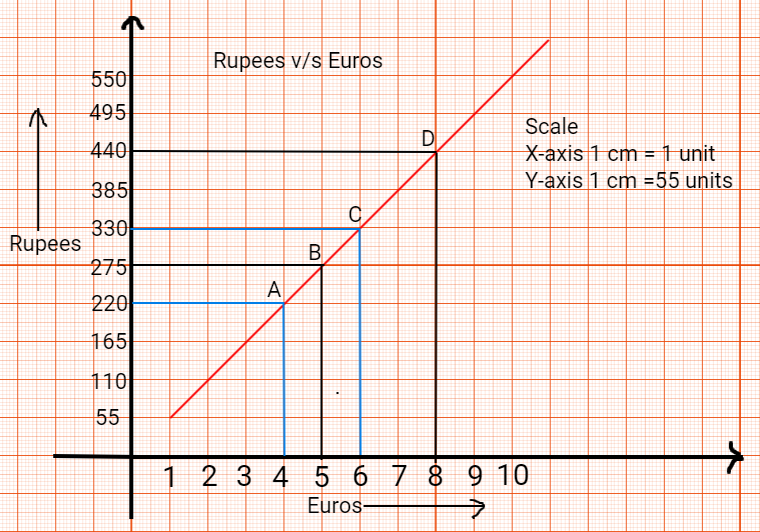QuestionAnswers

# On a particular day the exchange rate of 1 Euro was Rs. 55. The following linear Graph shows the relationship between the two currencies. Read the graph carefully. and answer the questions given below:(i) Find the values of 4 Euros in terms of Rupees.(ii) Find the values of 6 Euros in terms of Rupees.(iii) Find the value of Rs. 275 in terms of Euros.(iv) Find the value of Rs. 440 in terms of Euros.- Hint: Start by preparing the x and y axes. Plot Rupees on y-axis and Euros on x-axis with some assumed scale. Draw the linear variation of the exchange rate, Draw horizontal and vertical lines as per the conversion of Rupees to Euros or vice-versa.

(i). Draw a vertical line at x=4 parallel to y-axis intersecting the graph at A and then draw a horizontal line from this point towards y-axis and parallel to x-axis. The value found is Rs 220/- .
(ii). Draw a vertical line at x=6 parallel to y-axis intersecting the graph at B and then draw a horizontal line from this point towards y-axis and parallel to x-axis. The value found is Rs 330/- .
(iii). Draw a horizontal line at y=275 parallel to x-axis intersecting the graph at C and then draw a vertical line from this point towards x-axis and parallel to y-axis. The value found is € 5.
(iv). Draw a horizontal line at y=440 parallel to x-axis intersecting the graph at D and then draw a vertical line from this point towards x-axis and parallel to y-axis. The value found is € 8 .Note: Ruler and pencil should be used for drawing such graphs. One must mention the scale chosen to draw the graph over the upper right corner and name the graph .

View Notes
Measuring the Rate of Change of MotionStudy of Pollen Germination on a SlideThe Making of a ScientistChanging the Period of a PendulumGraphs of Trignometric FunctionsCBSE Class 10 Maths Chapter 1 - Real Numbers FormulaArea of a Sector of a Circle FormulaReflection on A Plane MirrorClass 10 IMO Maths Olympiad Sample Question Paper - 1IMO Sample Papers for Class 10Important Questions for CBSE Class 6 English A Pact with The Sun Chapter 1 - A Tale of Two BirdsImportant Questions for CBSE Class 11 Indian Economic Development Chapter 1 - Indian Economy on the Eve of IndependenceImportant Questions for CBSE Class 11 Accountancy Chapter 8 - Bill Of ExchangeImportant Questions for CBSE Class 11 Biology Chapter 17 - Breathing and Exchange of GasesImportant Questions for CBSE Class 10 Maths Chapter 1 - Real NumbersImportant Questions for CBSE Class 6 English A Pact with The Sun Chapter 10 - A Strange Wrestling MatchImportant Questions for CBSE Class 7 English Honeycomb Chapter 10 - The Story of CricketImportant Questions for CBSE Class 8 Science Chapter 10 - Reaching The Age of AdolescenceImportant Questions for CBSE Class 11 English Snapshots Chapter 1 - The Summer of the Beautiful White HorseCBSE Class 10 Hindi A Question Paper 2020Hindi A Class 10 CBSE Question Paper 2009Hindi A Class 10 CBSE Question Paper 2015Hindi A Class 10 CBSE Question Paper 2016Hindi A Class 10 CBSE Question Paper 2012Hindi A Class 10 CBSE Question Paper 2010Hindi A Class 10 CBSE Question Paper 2007Hindi A Class 10 CBSE Question Paper 2013Hindi A Class 10 CBSE Question Paper 2008Hindi A Class 10 CBSE Question Paper 2014RS Aggarwal Class 10 Solutions - T-Ratios of Some Particular AnglesRD Sharma Class 10 Solutions Chapter 1 - Real Numbers (Ex 1.1) Exercise 1.1RS Aggarwal Class 10 Solutions Chapter 1 - Real Numbers (Ex 1A) Exercise 1.1NCERT Solutions for Class 11 Biology Chapter 17NCERT Solutions for Class 10 Maths Chapter 1 Real Numbers (Ex 1.1) Exercise 1.1HC Verma Class 11 Physics Part-1 Solutions for Chapter 15 - Wave Motion and Waves on a StringRS Aggarwal Solutions Class 10 Chapter 10 - Quadratic Equations (Ex 10A) Exercise 10.1RD Sharma Class 10 Solutions Chapter 10 - Exercise 10.1RD Sharma Class 10 Solutions Chapter 1 - Real Numbers (Ex 1.2) Exercise 1.2RS Aggarwal Solutions Class 10 Chapter 1 - Real Numbers (Ex 1B) Exercise 1.2